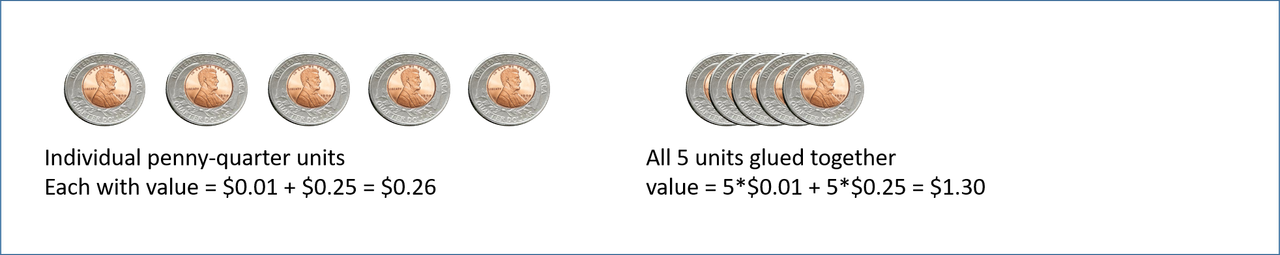June 07, 2020, 03:21:17 AM
Forum Rules: Read This Before Posting

###Topic: I can't understand molecular and empirical formula  (Read 156 times)

0 Members and 1 Guest are viewing this topic.

####Kago

• Very New Member
•• Posts: 1
• Mole Snacks: +0/-0##### I can't understand molecular and empirical formula
« on: May 19, 2020, 10:59:41 AM »
I'll use this problem as an example:

"A compound consists of of 26.67 carbon, 71.11 oxygen, 2.22 hydrogen per mass. If the relative formula mass of the compound is equal to 90, calculate the molecular (actual) formula of the compound."

The empirical formula is then disovered to be CO2H which shows the simplest ratio of elements in the compound. The empirical mass of it will then be calculated as 45 g/mol. So then to get the ratio in the molecular mass which is 90 you double each number in the element ratio by two.

This is what I don't get.

Why does the atom number double from the empirical mass to get the molecular mass? If I pour more of a substance to get more moles of it, the element number in the fixed ratio doesn't suddenly double per molecule, you only increase the compound number with elements in the same original ratio until you get 90g for the molecular mass.

So the ratio of elements should stay the same if mass increases right?

I think there's something I'm misunderstanding here. Hopefully someone can help clear this up.

####AWK

• Retired Staff
• Sr. Member
•• Posts: 7331
• Mole Snacks: +515/-86
• Gender:##### Re: I can't understand molecular and empirical formula
« Reply #1 on: May 19, 2020, 11:21:53 AM »
Try to draw the correct organic formula with the total formula CHO2 or C3H3O6. And C2H2O4 you can.
AWK

####Borek##### Re: I can't understand molecular and empirical formula
« Reply #2 on: May 19, 2020, 03:55:21 PM »
Take a look at simple carbohydrates - all tetroses have a molecular formula of (CH2O)4, all pentoses are (CH2O)5, all hexoses are (CH2O)6. Empirical formula of all of them is CH2O, yet molecular formulas are different.
ChemBuddy chemical calculators - stoichiometry, pH, concentration, buffer preparation, titrations.info, pH-meter.info

####MNIO

• Regular Member
•• Posts: 93
• Mole Snacks: +12/-1##### Re: I can't understand molecular and empirical formula
« Reply #3 on: May 19, 2020, 05:16:49 PM »
There's a difference between molecular and empirical formulas that you're not understanding
(1) the molecular formula represents all the atoms in the molecule
(2) the empirical formula represents the lowest whole number ratio of
atoms of different elements in a molecule
again,
1 represents the entire molecule
the other represents the lowest whole number ratio of different elements in the molecule

example the molecule H-O-O-H
molecular formula = H2O2
empirical formula = HO
there are 2 empirical units in every 1 molecule of H-O-O-H
the ratios of H:O are the same in both formulas right?  2:2 = 1:1 ?
but 1 (the molecular formula) indicates the smallest particle of that chemical
and the other represents the lowest whole number ratio of atoms of the 2 elements

example C6H12O6... glucose.. this molecule
https://en.wikipedia.org/wiki/Glucose#/media/File:D-glucose-chain-2D-Fischer.png
molecular formula = C6H12O6
empirical formula = CH2O... the lowest whole number ratios of C:H:O in the molecule 1:2:1
there are 6 empirical units per 1 molecule of glucose

*******
let's try another example
let's say I glue 1 penny to 1 quarter.  Then I repeat it 5 times.  So I have 5 penny-quarter units.
then let's say I glue all 5 penny-quarter units together into 1 lump.  see the attached picthe total lump is 5 Pennies + 5 Quarters.... let's write that as P5Q5
the lowest whole number ratio of P to Q represents the individual units  P1Q1
the lump has "lump value" = $1.30 the individual units have value =$0.26
if I divide $1.30 /$0.26  I get the value "5" meaning there are 5 units in the lump
I can multiple the units x 5 to get the "lump".  P1Q1 * 5 = P5Q5

********
now let's take this 1 step further.  let's say we glue some quarters, dimes, and pennies together
to make a lump with value approximately = $4.00 If we run an analysis, and I tell you that 50.0% of the coins are dimes, 16.7% are quarters and 33.3% are pennies, I could do this: (1) assume 100 coins (2) convert percents to coins (3) simply the ratios of the coins to the smallest unit (empirical coin unit) (4) divide the total value of the lump by empirical unit value to get # empirical units in any given lump. (5) multiply empirical unit by number of empirical units in the lump to the the formula for the lump. the "empirical unit has" # dimes = 100 coins * (0.500 dimes / coin) = 50 dimes # quarters = 100 * 0.093 = 16.7 quarters # pennies = 100 * 0.349 = 33.3 pennies now that's not whole numbers (it's hard to glue together 2/3 of a quarter and 1/3 of a penny right?) So lets find the lowest whole number ratio of those coins. We start by dividing all by whichever is the least # dimes = 50.0 / 16.7 = 3 # quarters = 16.7 / 16.7 = 1 # pennies = 33.3 / 16.7 = 2 and the "empirical" unit is P2QD3 with value = 2*0.01 + 1*0.25 + 3*0.10 =$0.57
the number of empirical units in our lump = $4.00 /$0.57 = 7
the formula of the lump is then 7*P2QD3 = P14Q7D21

*******
it's the same thing with molecules.. C6H126 with "mass" = 180
lowest ratio = CH2O with mass = 30
so the number of CH2O per molecule = 180 / 30 = 6
and I can write CH2O * 6 = C6H12O6

« Last Edit: May 19, 2020, 06:09:34 PM by MNIO »